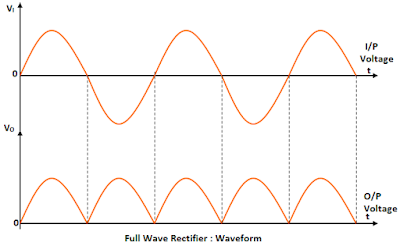# Full Wave Rectifier

The figure below shows the circuit diagram of a Full wave rectifier;The full wave rectifier contains diode D1 & D2. These diodes are connected in the centre tapped secondary coil of a transformer and a load resistance load RL.

An AC source is given in the primary coil of the transformer. Notice that only one – half the total secondary voltage is used for each diode.

Let V=Vm Sin ωt be the instantaneous sinusoidal voltage f=50 HZ appearing across the secondary coil, The secondary voltage is split into two halves one half is appearing across the diode D1 in series with RL, the other half appearing across the diode D2. This is also in series with the resistor load RL.Half Wave RectifierDuring the positive half cycle of the AC input, the terminal A is positive with respect to B. The diode  Dis forward biased. The current therefore flows through the diode D1 and voltage is developed across the load resistance RL. The direction of the current is indicated by solid arrows.

Now during the negative half cycle, the terminal A is negative with respect to B. The diode D2 is forward biased. Consequently, the current flows through the load resistor RLand is indicated by the dotted arrows.

Note that the rectified current flows in the same direction through RL during conduction on either diode. The diodes D1 & D2 conduct alternatively and both halves of the input appear across load. Hence the circuit functions as a full wave rectifier. The DC output voltage is positive of the common cathodes of the diodes.

The waveform's of the AC input voltage and the DC output voltage are shown in the fig. The rectified voltage is unidirectional, continuous but not constant.VI Characteristic of PN Junction Diode

### Peak Inverse Voltage:

Each diode in a full – wave rectifier is alternatively forward biased and reverse biased. When the diode D1 is forward biased the voltage across the non conduction  diode D2 is equal to sum of the voltage across the lower half of the secondary coil and the load resistor, i.e. maximum diode voltage VD2 = Vm – (-Vm) =2Vm

Hence peak inverse voltage across the non conducting diode is given by : PIV = 2VM.

### Ripple factor:

The ripple factor for the full wave rectifier is given by:
Ripple Factor = (rms value of AC component) / ( DC component)
= 0.482

### Efficiency of Rectification:

The rectifier efficiency is given by:
Rectifier Efficiency = Output DC power / Input AC power
= PDC / PAC  ~  81.2%

### Merits:

• When compared to half wave rectifier the output voltage is continuous

### Demerits:

• It is difficult to locate the centre tap on the secondary winding.
• The diode used must have high PIV
• The DC output is small as each diode utilizes only one-half of the transformer secondary voltage.
• Transformer with centre–tap is required.This post first appeared on My Tech Info, please read the originial post: here